# Exponential growth and decay word problems | Algebra II | Khan Academy

##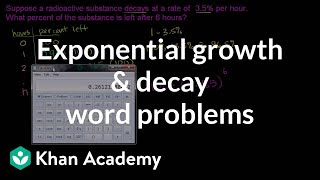By Khan Academy

Watch these examples of constructing exponential growth and decay models from the description of a relationship between two variables.# Properties of Rational Exponents Part Two

##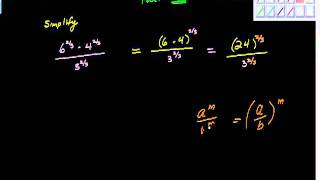By charlie Lindelof

YouTube presents Properties of Rational Exponents Part Two, an educational video resource on mathematics.# Solving an Equation Involving Rational Exponents - Example 2

##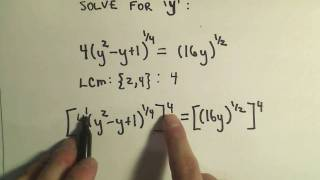By PatrickJMT

YouTube presents Solving an Equation Involving Rational Exponents - Example 2, an educational video resource on math.# Solving an Equation Involving Rational Exponents - Example 3

##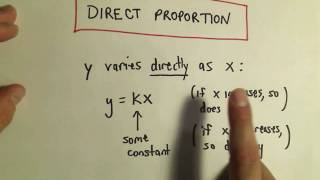By PatrickJMT

YouTube presents Solving an Equation Involving Rational Exponents - Example 3, an educational video resource on math.# Properties of Rational Exponents

##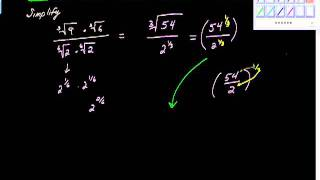By charlie Lindelof

YouTube presents Properties of Rational Exponents, an educational video resource on mathematics.# More on Rational Exponents

##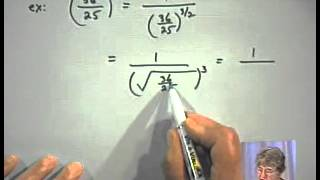By Learners TV

More on Rational Exponents# Solving an Equation Involving Rational Exponents - Example 1

##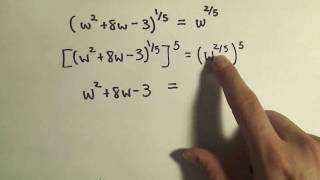By PatrickJMT

Youtube Presents Solving an Equation Involving Rational Exponents - Example 1 an educational video resources on english language arts# "Rational Exponents" | Algebra 1 with Educator.com

##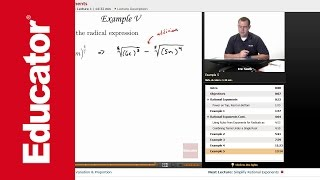By Educator

"Rational Exponents" | Algebra 1 with Educator.com# Definition, Evaluation of numbers with Rational Exponents

##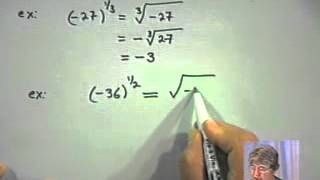By Learners TV

Definition, Evaluation of numbers with Rational Exponents# Simplify Rational Exponents

##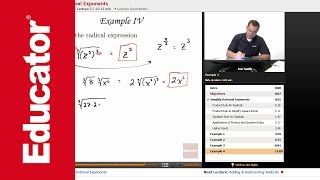By Educator

Simplify Rational Exponents# Exponents: Multiplying Variables with Rational Exponents – Basic Ex 2

##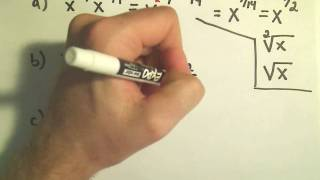By PatrickJMT

Exponents: Multiplying Variables with Rational Exponents – Basic Ex 2# Algebra 2 - Rational Exponents

##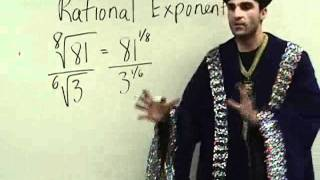By Yaymath

Join the mathemagician's adventure into simplifying expressions with different exponents. Fraction exponents, negative exponents, and roots of varying powers. YAY MATH!# Ex: Evaluate an Expression with Rational Exponents Using Radicals

##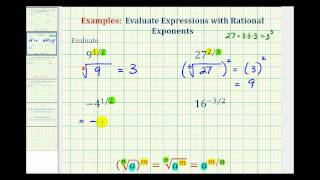By Mathispower4u

This video provides examples of how to evaluate an expressions with rational exponents by writing the expression in radical form and then simplifying.# Simplifying a radical expression using rational exponents

##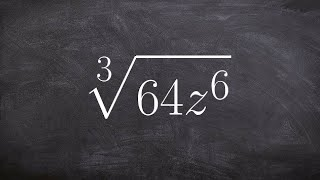By Brain McLogan

Learn how to simplify rational powers using the power and the product rules. There are some laws of exponents which might come handy when simplifying expressions with exponents. Some of the laws include the product law which states that the product of numbers/expressions having the same base is equivalent to taking one of the base with the sum of the original exponents as the new exponent. The power law says that when an expression with an exponent is raised to another exponent that it is equivalent to the expression with the product of the original exponents as the new exponent. Knowledge of these exponent laws will help you in simplifying power expressions using the power rule and the quotient rule.# Exponents: Multiplying Variables with Rational Exponents – Basic Ex 1

##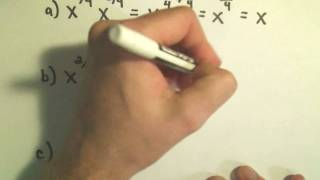By PatrickJMT

Exponents: Multiplying Variables with Rational Exponents – Basic Ex 1# More rational exponents and exponent laws | Algebra I | Khan Academy

##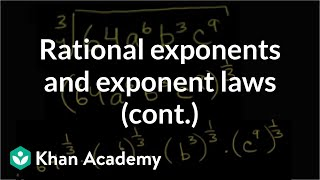By Khan Academy

Khan Academy presents More Rational Exponents and Exponent Laws, an educational video resource on math.# Radical equivalent to rational exponents 2 | Algebra I | Khan Academy

##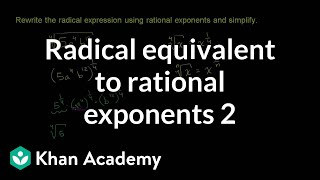By Khan Academy

Khan Academy presents Radical Equivalent to Rational Exponents 2, an educational video resource on math.# Radical equivalent to rational exponents | Algebra I | Khan Academy

##By Khan Academy

Radical Equivalent to Rational Exponents# Simplify Rational Exponents" | Algebra 1 with Educator.com

##By Educator

Simplify Rational Exponents" | Algebra 1 with Educator.com# Multi-step simplification of rational exponent expressions

##By Khan Academy

Sal shows how to rewriteï¿½ï¿½ï¿½ï¿½ï¿½ï¿½ï¿½ï¿½ï¿½ï¿½ï¿½ï¿½ï¿½ï¿½ï¿½ï¿½ï¿½ï¿½ï¿½ï¿½ï¿½ï¿½ï¿½ï¿½ï¿½ï¿½ï¿½ï¿½ï¿½ï¿½(5a^4b^12) as an exponential expression and then simplify it.